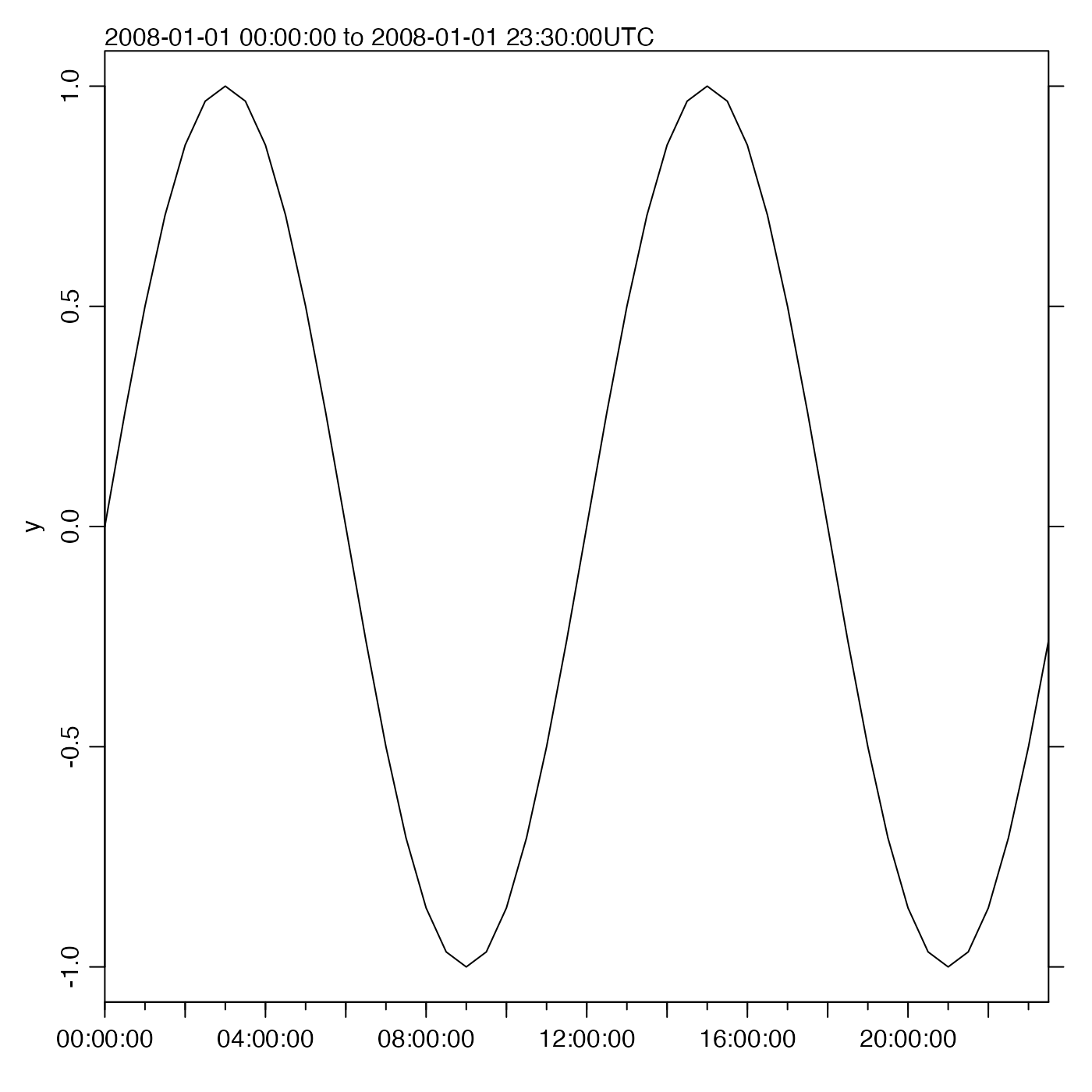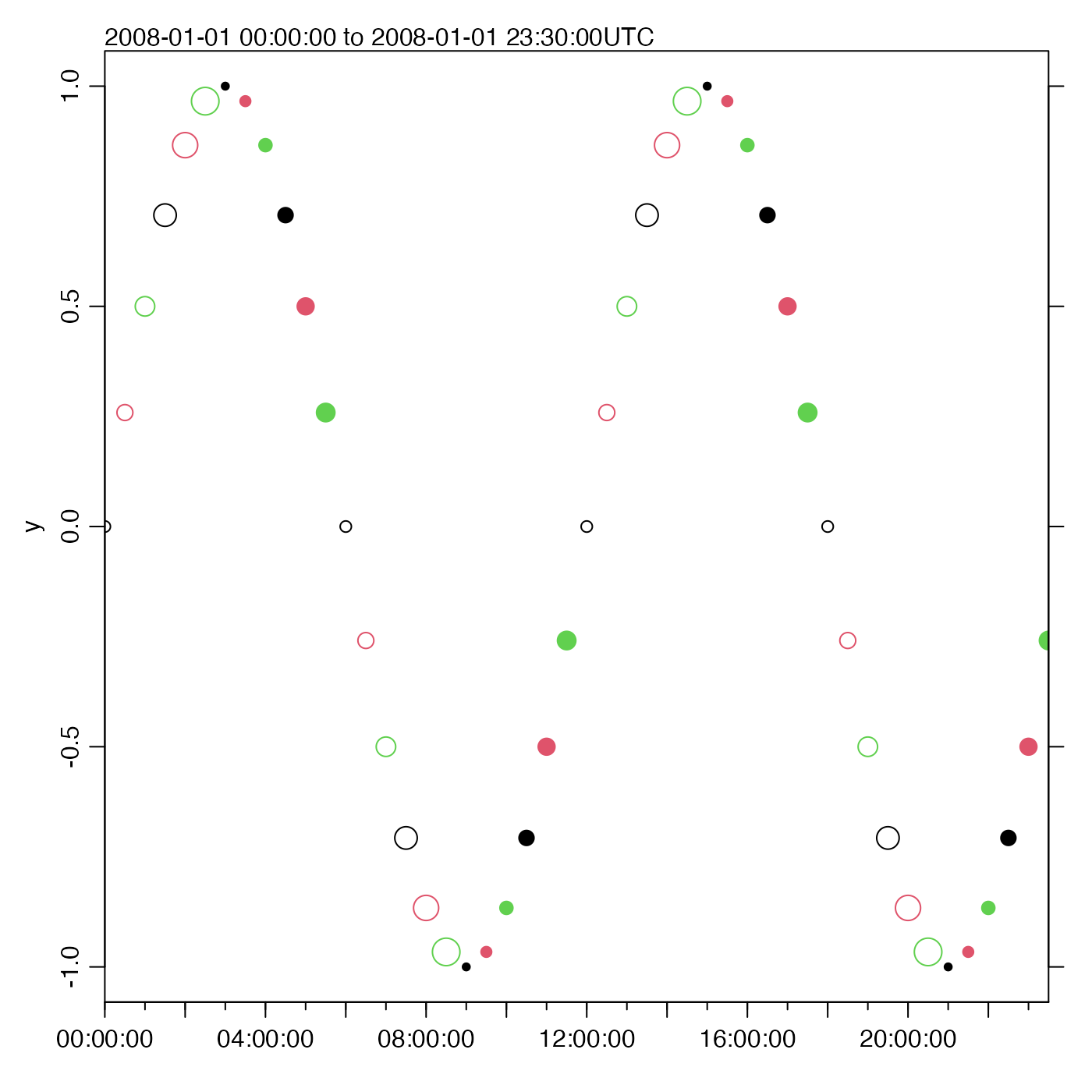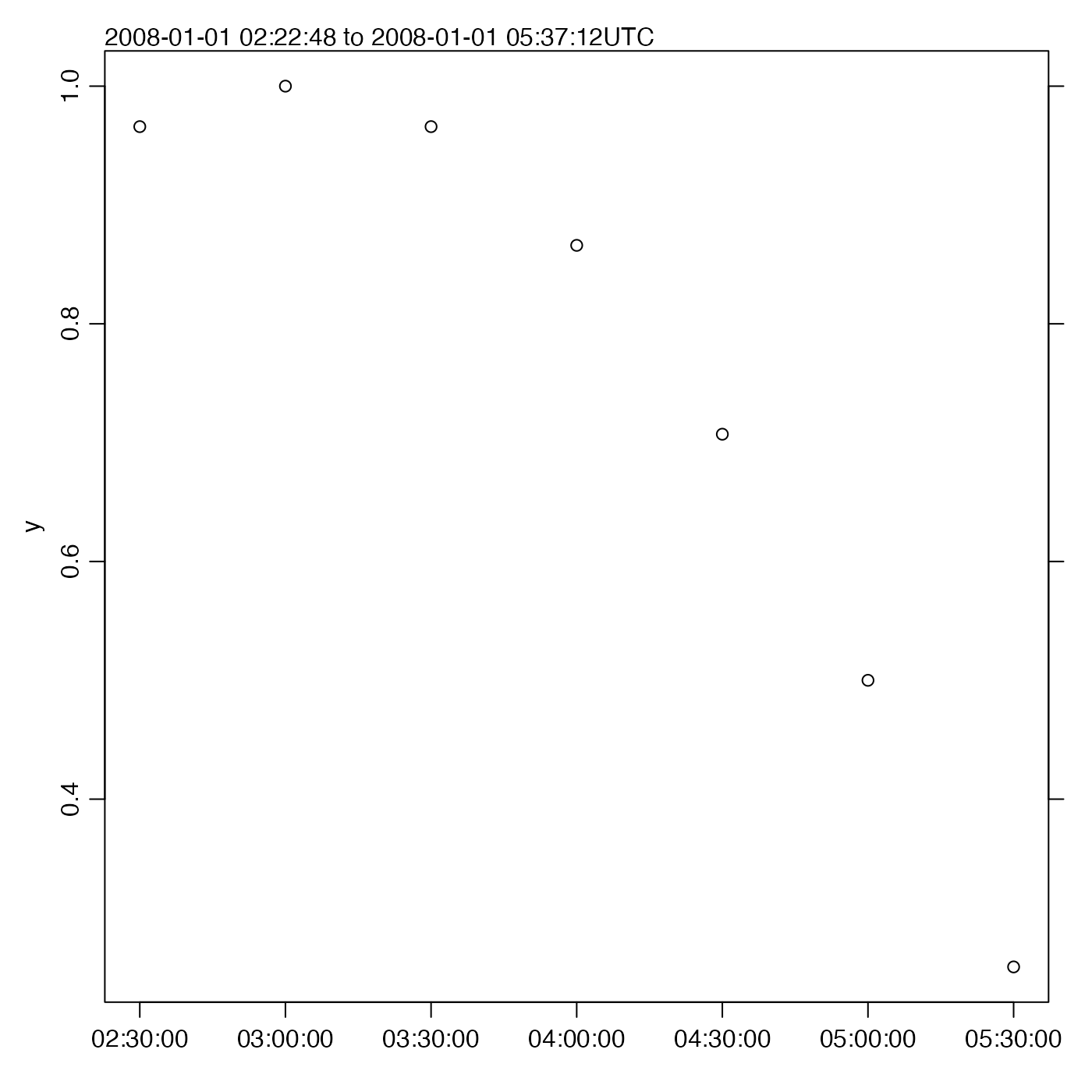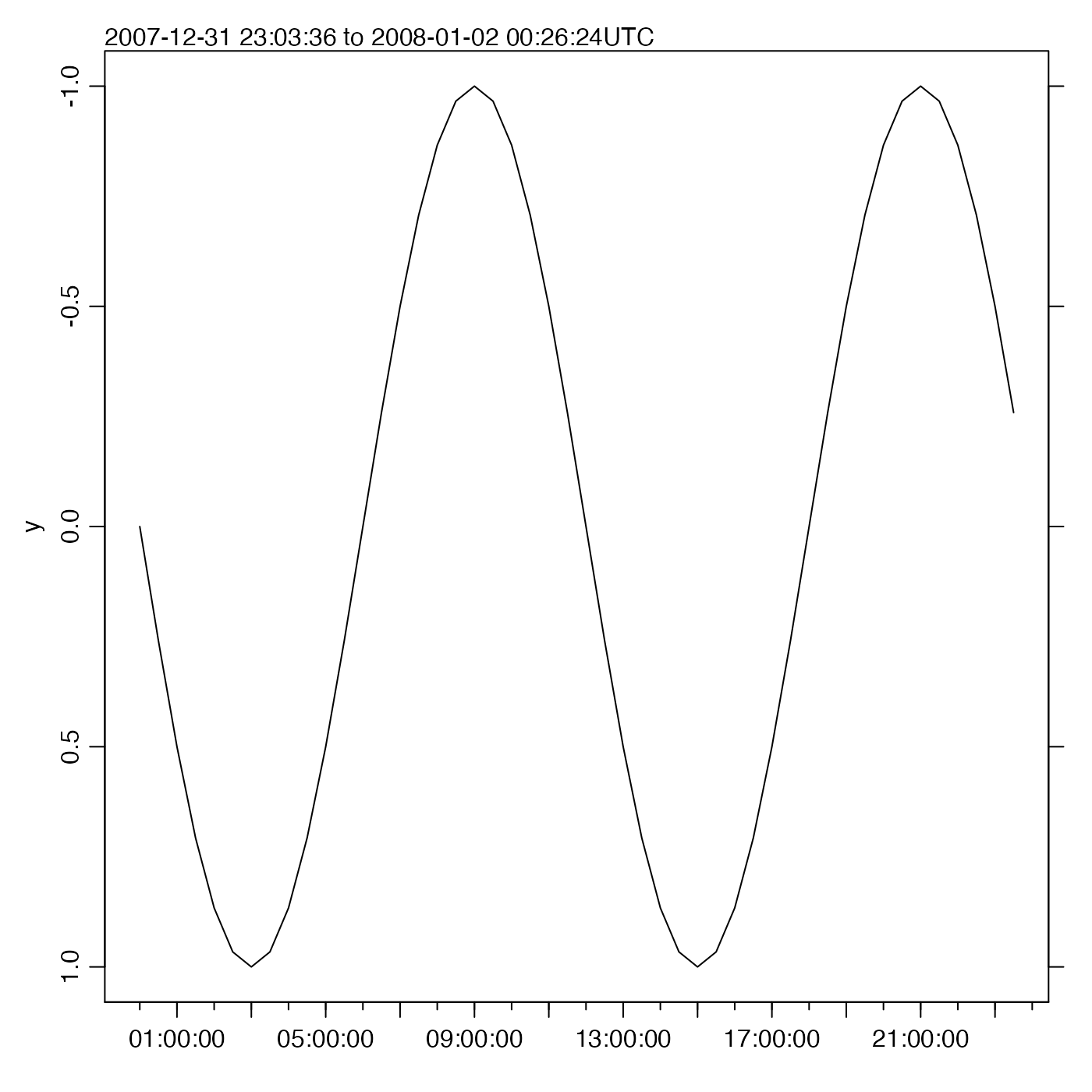Plot a time-series, obeying the timezone and possibly drawing the range in the top-left margin.

oce.plot.ts(
x,
y,
type = "l",
xlim,
ylim,
log = "",
logStyle = "r",
flipy = FALSE,
xlab,
ylab,
drawTimeRange,
simplify = 2560,
fill = FALSE,
col = par("col"),
pch = par("pch"),
cex = par("cex"),
cex.axis = par("cex.axis"),
cex.lab = par("cex.lab"),
cex.main = par("cex.main"),
xaxs = par("xaxs"),
yaxs = par("yaxs"),
mgp = getOption("oceMgp"),
mar = c(mgp + if (nchar(xlab) > 0) 1.5 else 1, mgp + 1.5, mgp + 1, mgp +
3/4),
main = "",
despike = FALSE,
axes = TRUE,
tformat,
marginsAsImage = FALSE,
grid = FALSE,
grid.col = "lightgray",
grid.lty = "dotted",
grid.lwd = par("lwd"),
debug = getOption("oceDebug"),
...
)

## Arguments

x

the times of observations. If this is not a POSIXt object, then an attempt is made to convert it to one using as.POSIXct().

y

the observations.

type

plot type, "l" for lines, "p" for points.

xlim

optional limit for x axis. This has an additional effect, beyond that for conventional R functions: it effectively windows the data, so that autoscaling will yield limits for y that make sense within the window.

ylim

optional limit for y axis.

log

a character value that must be either empty (the default) for linear y axis, or "y" for logarithmic y axis. (Unlike plot.default() etc., oce.plot.ts does not permit logarithmic time, or x axis.)

logStyle

a character value that indicates how to draw the y axis, if log="y". If it is "r" (the default) then the conventional R style is used, in which a logarithmic transform connects y values to position on the "page" of the plot device, so that tics will be nonlinearly spaced, but not organized by integral powers of 10. However, if it is "decade", then the style will be that used in the scientific literature, in which large tick marks are used for integral powers of 10, with smaller tick marks at integral multiples of those powers, and with labels that use exponential format for values above 100 or below 0.01. The value of logStyle is passed to oceAxis(), which draws the axis.

flipy

Logical, with TRUE indicating that the graph should have the y axis reversed, i.e. with smaller values at the bottom of the page.

xlab

name for x axis; defaults to "".

ylab

name for y axis; defaults to the plotted item.

drawTimeRange

an optional indication of whether/how to draw a time range, in the top-left margin of the plot; see oce.axis.POSIXct() for details.

simplify

an integer value that indicates whether to speed up type="l" plots by replacing the data with minimum and maximum values within a subsampled time mesh. This can speed up plots of large datasets (e.g. by factor 20 for 10^7 points), sometimes with minor changes in appearance. This procedure is skipped if simplify is NA or if the number of visible data points is less than 5 times simplify. Otherwise, oce.plot.ts creates simplify intervals ranging across the visible time range. Intervals with under 2 finite y data are ignored. In the rest, y values are replaced with their range, and x values are replaced with the repeated midpoint time. Thus, each retained sub-interval has exactly 2 data points. A warning is printed if this replacement is done. The default value of simplify means that cases with under 2560 visible points are plotted conventionally.

fill

boolean, set TRUE to fill the curve to zero (which it does incorrectly if there are missing values in y).

col

The colours for points (if type=="p") or lines (if type=="l"). For the type="p" case, if there are fewer col values than there are x values, then the col values are recycled in the standard fashion. For the type="l" case, the line is plotted in the first colour specified.

pch

character code, used if type=="p". If there are fewer pch values than there are x values, then the pch values are recycled in the standard fashion. See points() for the possible values for pch.

cex

numeric character expansion factor for points on plots, ignored unless type is "p". This may be a single number, applied to all points, or a vector of numbers to be applied to the points in sequence. If there are fewer pch values than there are x values, then the pch values are recycled in the standard fashion. See par() for more on cex.

cex.axis, cex.lab, cex.main

numeric character expansion factors for axis numbers, axis names and plot titles; see par().

xaxs

control x axis ending; see par("xaxs").

yaxs

control y axis ending; see par("yaxs").

mgp

3-element numerical vector to use for par(mgp), and also for par(mar), computed from this. The default is tighter than the R default, in order to use more space for the data and less for the axes.

mar

value to be used with par("mar") to set margins. The default value uses significantly tighter margins than is the norm in R, which gives more space for the data. However, in doing this, the existing par("mar") value is ignored, which contradicts values that may have been set by a previous call to drawPalette(). To get plot with a palette, first call drawPalette(), then call oce.plot.ts with mar=par("mar").

main

title of plot.

despike

boolean flag that can turn on despiking with despike().

axes

boolean, set to TRUE to get axes plotted

tformat

optional format for labels on the time axis

marginsAsImage

boolean indicating whether to set the right-hand margin to the width normally taken by an image drawn with imagep().

grid

if TRUE, a grid will be drawn for each panel. (This argument is needed, because calling grid() after doing a sequence of plots will not result in useful results for the individual panels.

grid.col

color of grid

grid.lty

line type of grid

grid.lwd

line width of grid

debug

a flag that turns on debugging. Set to 1 to get a moderate amount of debugging information, or to 2 to get more.

...

graphical parameters passed down to plot().

## Value

A list is silently returned, containing xat and yat, values that can be used by oce.grid() to add a grid to the plot.

## Details

Depending on the version of R, the standard plot() and plot.ts() routines will not obey the time zone of the data. This routine gets around that problem. It can also plot the time range in the top-left margin, if desired; this string includes the timezone, to remove any possible confusion. The time axis is drawn with oce.axis.POSIXct().

## Author

Dan Kelley and Clark Richards

## Examples

library(oce)
t0 <- as.POSIXct("2008-01-01", tz="UTC")
t <- seq(t0, length.out=48, by="30 min")
y <- sin(as.numeric(t - t0) * 2 * pi / (12 * 3600))
oce.plot.ts(t, y, type='l', xaxs='i')# Show how col, pch and cex get recycled
oce.plot.ts(t, y, type='p', xaxs='i',
col=1:3, pch=c(rep(1, 6), rep(20, 6)), cex=sqrt(1:6))# Trimming x; note the narrowing of the y view
oce.plot.ts(t, y, type='p', xlim=c(t, t))# Flip the y axis
oce.plot.ts(t, y, flipy=TRUE)